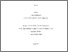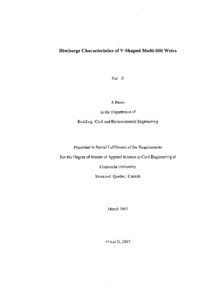Title:

# Discharge characteristics of V-shaped multi-slit weirs

Ji, Kai (2007) Discharge characteristics of V-shaped multi-slit weirs. Masters thesis, Concordia University.Preview Text (application/pdf) MR28901.pdf - Accepted Version 2MB

## Abstract

V-shaped weirs with small angles are efficient in accurately measuring extremely small discharge rates. The concept of slit weirs to measure discharge rates has been studied in recent investigations. Rectangular multi-slit notches or weirs have been shown to extend the range of flow measurement to include both very low and high discharge rates. It is a well-known fact that V-notches whose sections are narrower at lower heads are preferred to rectangular notches to measure small discharge rates in laboratories. To increase the range of discharges measured, the concept of multi-slit weirs using rectangular weirs has been introduced recently. For rectangular multi-slit weirs, experimental data showed that the discharge coefficient C d for multi-slit weirs or a single weir was a function of the Reynolds number R e when R e is based on the width of rectangular weirs. V-notches are generally used for laboratory flow measurements, as they can very precisely measure discharge rates. Hence, the multi-slit weirs formed by V-notches are expected to measure discharges in a very wide range of discharge rates. To represent a common discharge coefficient C d for multi-slit weirs formed of either rectangular or triangular shaped individual weirs, a new Reynolds number R eR is proposed to unify representation of discharge coefficient data for both rectangular shaped and V-shaped multi-slit weir units. C d data for both rectangular and V-shaped multi-slit weirs fall on a single curve and appear to be the function of R eR . At large Reynolds number, R eR , the discharge coefficient C d approaches a constant value of 0.61. This constant also denotes the contraction coefficient for the narrow two-dimensional slit flow. Present test data show that C d for multi-slit V-shaped weirs is generally a function of R eR for b/B <0.3. Here, R eR is based on the hydraulic radius R and b is the value of top flow width of the water passing the multi-slit weirs. A very limited number of numerical simulations are also performed to determine discharge coefficient ( C d ) and the water surface profiles for the multi-slit V-shaped weirs. The predictions related to the surface profile based on the numerical model are validated by experimental data. The three-dimensional (3D) two-equation standard k- [varepsilon] turbulence model together with the volume of fluid VOF tracking technique is effectively used to obtain a few simulation results that are limited in scope

Divisions: Concordia University > Gina Cody School of Engineering and Computer Science > Building, Civil and Environmental Engineering Thesis (Masters) Ji, Kai xiii, 82 leaves : ill. ; 29 cm. Concordia University M.A. Sc. Building, Civil and Environmental Engineering 2007 Ramamurthy, Amruthur LE 3 C66B85M 2007 J5 975256 Concordia University Library 22 Jan 2013 16:04 13 Jul 2020 20:07 https://concordiauniversity.on.worldcat....
All items in Spectrum are protected by copyright, with all rights reserved. The use of items is governed by Spectrum's terms of access.

Repository Staff Only: item control pageResearch related to the current document (at the CORE website)
Back to top